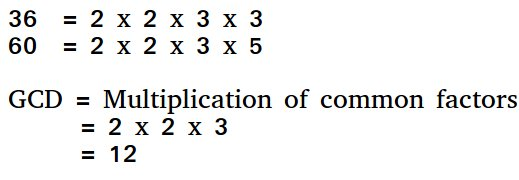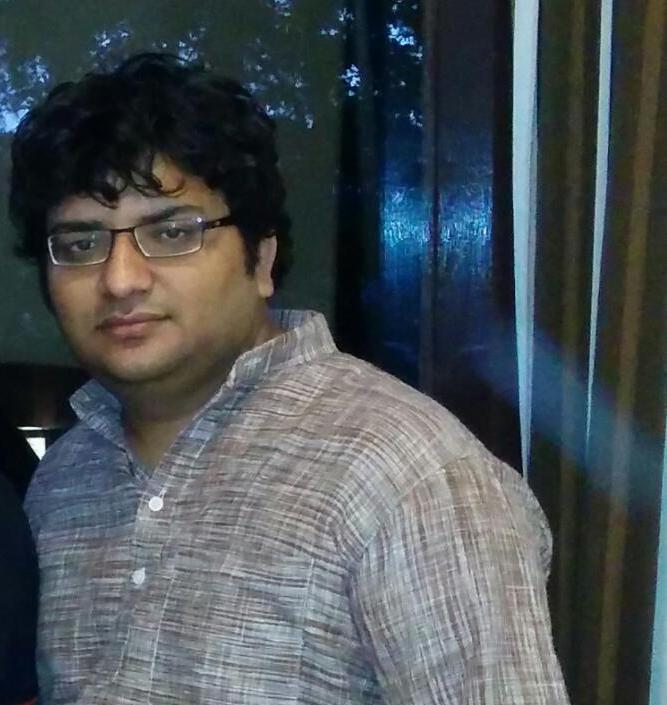# Program to find HCF (Highest Common Factor) of 2 Numbers

HCF (Highest Common Factor) or GCD (Greatest Common Divisor) of two numbers is the largest number that divides both of them.For example GCD of 20 and 28 is 4 and GCD of 98 and 56 is 14.

## Recommended: Please solve it on “PRACTICE ” first, before moving on to the solution.

A simple solution is to find all prime factors of both numbers, then find intersection of all factors present in both numbers. Finally return product of elements in the intersection.

An efficient solution is to use Euclidean algorithm which is the main algorithm used for this purpose. The idea is, GCD of two numbers doesn’t change if smaller number is subtracted from a bigger number.

## C

 `// C program to find GCD of two numbers ` `#include ` ` `  `// Recursive function to return gcd of a and b ` `int` `gcd(``int` `a, ``int` `b) ` `{ ` `    ``// Everything divides 0  ` `    ``if` `(a == 0 || b == 0) ` `       ``return` `0; ` ` `  `    ``// base case ` `    ``if` `(a == b) ` `        ``return` `a; ` ` `  `    ``// a is greater ` `    ``if` `(a > b) ` `        ``return` `gcd(a-b, b); ` `    ``return` `gcd(a, b-a); ` `} ` ` `  `// Driver program to test above function ` `int` `main() ` `{ ` `    ``int` `a = 98, b = 56; ` `    ``printf``(``"GCD of %d and %d is %d "``, a, b, gcd(a, b)); ` `    ``return` `0; ` `} `

## Java

 `// Java program to find GCD of two numbers ` `class` `Test ` `{ ` `    ``// Recursive function to return gcd of a and b ` `    ``static` `int` `gcd(``int` `a, ``int` `b) ` `    ``{ ` `        ``// Everything divides 0  ` `        ``if` `(a == ``0` `|| b == ``0``) ` `           ``return` `0``; ` `      `  `        ``// base case ` `        ``if` `(a == b) ` `            ``return` `a; ` `      `  `        ``// a is greater ` `        ``if` `(a > b) ` `            ``return` `gcd(a-b, b); ` `        ``return` `gcd(a, b-a); ` `    ``} ` `     `  `    ``// Driver method ` `    ``public` `static` `void` `main(String[] args)  ` `    ``{ ` `        ``int` `a = ``98``, b = ``56``; ` `        ``System.out.println(``"GCD of "` `+ a +``" and "` `+ b + ``" is "` `+ gcd(a, b)); ` `    ``} ` `} `

## Python3

 `# Recursive function to return gcd of a and b ` `def` `gcd(a,b): ` `     `  `    ``# Everything divides 0  ` `    ``if` `(a ``=``=` `0` `or` `b ``=``=` `0``): ` `            ``False` `    ``# base case ` `    ``if` `(a ``=``=` `b): ` `        ``return` `a ` ` `  `    ``# a is greater ` `    ``if` `(a > b): ` `        ``return` `gcd(a``-``b, b) ` `    ``return` `gcd(a, b``-``a) ` ` `  `# Driver program to test above function ` `a ``=` `98` `b ``=` `56` `if``(gcd(a, b)): ` `    ``print``(``'GCD of'``, a, ``'and'``, b, ``'is'``, gcd(a, b)) ` `else``: ` `    ``print``(``'not found'``) ` ` `  `# This code is contributed by Danish Raza `

## C#

 `// C# program to find GCD of two ` `// numbers ` `using` `System; ` ` `  `class` `GFG { ` `     `  `    ``// Recursive function to return ` `    ``// gcd of a and b ` `    ``static` `int` `gcd(``int` `a, ``int` `b) ` `    ``{ ` `         `  `        ``// Everything divides 0  ` `        ``if` `(a == 0 || b == 0) ` `            ``return` `0; ` `     `  `        ``// base case ` `        ``if` `(a == b) ` `            ``return` `a; ` `     `  `        ``// a is greater ` `        ``if` `(a > b) ` `            ``return` `gcd(a - b, b); ` `             `  `        ``return` `gcd(a, b - a); ` `    ``} ` `     `  `    ``// Driver method ` `    ``public` `static` `void` `Main()  ` `    ``{ ` `        ``int` `a = 98, b = 56; ` `        ``Console.WriteLine(``"GCD of "`  `          ``+ a +``" and "` `+ b + ``" is "`  `                      ``+ gcd(a, b)); ` `    ``} ` `} ` ` `  `// This code is contributed by anuj_67. `

## PHP

 ` ``\$b``) ` `        ``return` `gcd( ``\$a``-``\$b` `, ``\$b` `) ; ` ` `  `    ``return` `gcd( ``\$a` `, ``\$b``-``\$a` `) ; ` `} ` ` `  `// Driver code ` `\$a` `= 98 ; ` `\$b` `= 56 ; ` ` `  `echo` `"GCD of \$a and \$b is "``, gcd(``\$a` `, ``\$b``) ; ` ` `  `// This code is contributed by Anivesh Tiwari ` `?> `

Output:

`GCD of 98 and 56 is 14`

A more efficient solution is to use modulo operator in Euclidean algorithm .

## C

 `// C program to find GCD of two numbers ` `#include ` ` `  `// Recursive function to return gcd of a and b ` `int` `gcd(``int` `a, ``int` `b) ` `{ ` `    ``if` `(b == 0) ` `        ``return` `a; ` `    ``return` `gcd(b, a % b);  ` `} ` ` `  `// Driver program to test above function ` `int` `main() ` `{ ` `    ``int` `a = 98, b = 56; ` `    ``printf``(``"GCD of %d and %d is %d "``, a, b, gcd(a, b)); ` `    ``return` `0; ` `} `

## Java

 `// Java program to find GCD of two numbers ` `class` `Test ` `{ ` `    ``// Recursive function to return gcd of a and b ` `    ``static` `int` `gcd(``int` `a, ``int` `b) ` `    ``{ ` `      ``if` `(b == ``0``) ` `        ``return` `a; ` `      ``return` `gcd(b, a % b);  ` `    ``} ` `     `  `    ``// Driver method ` `    ``public` `static` `void` `main(String[] args)  ` `    ``{ ` `        ``int` `a = ``98``, b = ``56``; ` `        ``System.out.println(``"GCD of "` `+ a +``" and "` `+ b + ``" is "` `+ gcd(a, b)); ` `    ``} ` `} `

## Python3

 `# Recursive function to return gcd of a and b ` `def` `gcd(a,b): ` `     `  `    ``# Everything divides 0  ` `    ``if` `(b ``=``=` `0``): ` `         ``return` `a ` `    ``return` `gcd(b, a``%``b) ` ` `  `# Driver program to test above function ` `a ``=` `98` `b ``=` `56` `if``(gcd(a, b)): ` `    ``print``(``'GCD of'``, a, ``'and'``, b, ``'is'``, gcd(a, b)) ` `else``: ` `    ``print``(``'not found'``) ` ` `  `# This code is contributed by Danish Raza `

## C#

 `// C# program to find GCD of two ` `// numbers ` `using` `System; ` ` `  `class` `GFG { ` `     `  `    ``// Recursive function to return ` `    ``// gcd of a and b ` `    ``static` `int` `gcd(``int` `a, ``int` `b) ` `    ``{       ` `       ``if` `(b == 0) ` `          ``return` `a; ` `       ``return` `gcd(b, a % b);  ` `    ``} ` `     `  `    ``// Driver method ` `    ``public` `static` `void` `Main()  ` `    ``{ ` `        ``int` `a = 98, b = 56; ` `        ``Console.WriteLine(``"GCD of "`  `          ``+ a +``" and "` `+ b + ``" is "`  `                      ``+ gcd(a, b)); ` `    ``} ` `} ` ` `  `// This code is contributed by anuj_67. `

## PHP

 ` `

Output:

`GCD of 98 and 56 is 14`

Please refer GCD of more than two (or array) numbers to find HCF of more than two numbers.

Attention reader! Don’t stop learning now. Get hold of all the important DSA concepts with the DSA Self Paced Course at a student-friendly price and become industry ready.

My Personal Notes arrow_drop_upCheck out this Author's contributed articles.

If you like GeeksforGeeks and would like to contribute, you can also write an article using contribute.geeksforgeeks.org or mail your article to contribute@geeksforgeeks.org. See your article appearing on the GeeksforGeeks main page and help other Geeks.

Please Improve this article if you find anything incorrect by clicking on the "Improve Article" button below.

Improved By : vigneshbabuvenkatesh

Article Tags :
Practice Tags :

Be the First to upvote.

Please write to us at contribute@geeksforgeeks.org to report any issue with the above content.